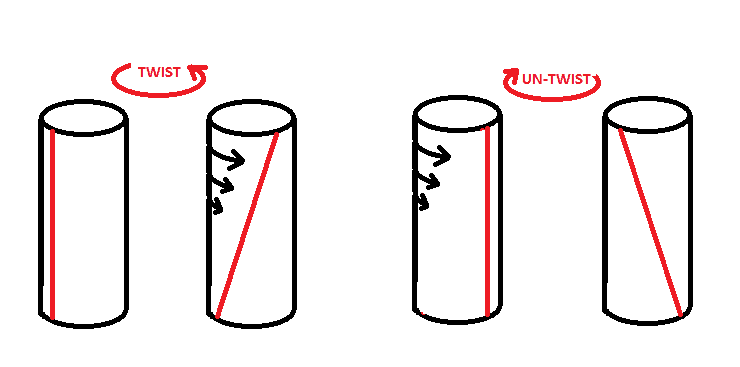# Gauge symmetry of cylindrical rod

kau
A twisted cylindrial rod has the cross sectional symmetry so that it's not posible to tell whether it is twisted or not without knowing if there is any torsional energy. now drawing a line on the surface of it can tell us whether or not it's twisted. It might not be a straight line.. there are infintes of such lines and any one of them would tell us the difference. could anyone explain what difference would it make if i draw a line on a untwisted surface and twisted one... though i got the idea mostly but having problem in visualization.
thanks

## Answers and Replies

A.R.
I hope I'll help. If you trace a line (for semplicity, a vertical, straight line, without lo ss of generality) on an un-twisted rod, and then you twist it (for example, leaving the bottom still and moving the top), supposing the twisting homogeneus, the line will be distorted like in the figure. If you trace the same line on the twisted rod, un-twisting it will cause the line to assume the same disposition of the previous line, but negative.In other words, if L1 and L2 are the lines drawn on the un-twisted and twisted rod respectively, and T and U are the twisting / un-twisting functions, then
T(L1) = - U(L2)

kau
I hope I'll help. If you trace a line (for semplicity, a vertical, straight line, without lo ss of generality) on an un-twisted rod, and then you twist it (for example, leaving the bottom still and moving the top), supposing the twisting homogeneus, the line will be distorted like in the figure. If you trace the same line on the twisted rod, un-twisting it will cause the line to assume the same disposition of the previous line, but negative.
View attachment 78048
In other words, if L1 and L2 are the lines drawn on the un-twisted and twisted rod respectively, and T and U are the twisting / un-twisting functions, then
T(L1) = - U(L2)[/
woud you explain the untwisting part.....why the line changes its allignment at the beginning of untwisting...that is you got a line alligned in right diagonal way but you start untwisting with it oriented towards left diagonal direction..

A.R.
In order to formalize what "twisting" is, let's divide the rod in slices, each one with same thickness. For example, each slice is thick as the distance between two consecutive black arrows. If you twist the rod moving only the top and leaving the bottom still, you are rotating the top slice of a certain angle θ=Θ, while the bottom slice is not rotating at all, i.e., for the bottom slice, the angle of rotation is θ=0. Each slice in between rotates of a certain angle between 0 and Θ. If the relation that describes θ along the axis is linear
θ(x)=mx, with x the number of the slice (x=0 indicates the bottom slice, x=1 the top one) and m a certain coefficient,
then the twisting is homogeneous.
If you twst the rod, and then trace the vertical line, each slice will be "marked" with a segment of the line. Un-twisting, each segment will rotate along with the slice of the same angle it (the slice) was twisted before, so slice and segment will rotate of a certain angle
θ(x)= - mx
where the minus sign implies that the rod is UN-twisting, so returning to it's previous state. You obtain than the same relation between angle and longitudinal coordinate, but negative. If you reduce the slice thickness near to zero, x becomes a real number and the line distorts continuously, following the same relation of θ.

EDIT: Plus, I thought it was obvious, the two parts of the picture are two different cases. You asked what would happen if one draws a line on an rod and on a twisted one. You start in both cases with a vertical line, then it distort in two symmetrical ways.

Last edited: